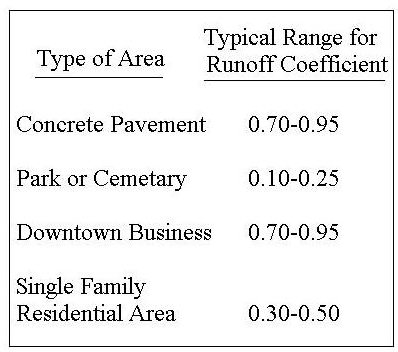# The Rational Method for Estimation of Design Surface Runoff Rate for Storm Water Control

Page content

## Introduction

The Rational Method is widely used to estimate the peak surface runoff rate for design of a variety of drainage structures, such as a length of storm sewer, a storm water inlet, or a storm water detention pond. The Rational Method is most suitable for small urban watersheds that don’t have storage such as ponds or swamps. It is best for areas less than 100 acres, but is sometimes used for up to 2 mi2 areas.

## The Rational Method Equation

The equation that is the centerpiece of the Rational Method is: q = CiA, where q is the peak surface runoff rate in cfs, from a watershed of area, A acres, and runoff coefficient, C, due to a storm of intensity, i in/hr. The units on peak runoff rate, q, are actually acre-in/hr, but the conversion from acre-in/hr to cfs is very nearly one, so the more common unit, cfs, is tyically used for q. In order to calculate a value for peak runoff rate for a given drainage area, values are needed for the three parameters, A, C, and i. Each of these parameters will be discussed separately in the next three sections.

## The Drainage Area, A

The drainage area, A, is often determined from a map which includes the drainage area of interest. It may be necessary to first determine the boundaries of the drainage area using a contour map. Once the boundaries are known, the area can be determined using the map scale. Since the area must be in acres for use in the Rational Method equation, a useful conversion factor is 43,560 ft2/acre. A map with a drainage area outlined is shown at the left.

## The Runoff Coefficient, C

The runoff coefficient is the fraction of rainfall striking the drainage area that becomes runoff from that drainage area. It is an empiricallydetermined constant, dependent on the nature of the drainage area surface. An impervious surface like a concrete parking lot will have a runoff coefficient of nearly one. A very tight clay soil will also have a relatively high runoff coefficient, while a sandy soil would have more infiltration and a lower runoff coefficient. In addition to the nature of the surface and the soil, the slope of the drainage area has an effect on the runoff coefficient. A greater slope leads to a higher runoff coefficient. There are many tables of values for runoff coefficient for a variety of types of drainage areas in handbooks, textbooks and on the internet. The table at the right shows some typical ranges of values for runoff coefficient.

The article, “Runoff Coefficients for Use in Rational Method Calculations.” more details on the runoff coefficient and a more comprehensive rational method runoff coefficient table of values.

## The Design Rainfall Intensity, i

The design rainfall intensity is the intensity of a constant intensity design storm with the specified design return period and duration equal to the time of concentration of the drainage area. Once the design return period and duration are determined, the design rainfall intensity can be determined from an appropriate intensity-duration-frequency graph or equation for the location of the drainage area. As you can see, determining a value for i is the most complicated part of using the Rational Method. The procedure for doing so is covered in a separate article, “Calculating Design Rainfall Intensity for Use in the Rational Method.”

## Example Calculation

Consider a section of a downtown business area of 35,400 square feet that drains to a particular storm water inlet. The runoff coefficient for this drainage area has been estimated to be 0.85. Based on a specified design return period and the time of concentration of the drainage area, the design storm intensity has been determined to be 5.1 in/hr. What is the peak runoff rate from this area to be used for design of the storm water inlet?

Solution: q = CiA = (0.85)(5.1)(35,400/43,560) cfs = 3.52 cfs.

One use for the Rational Method is in calculating design storm water runoff rate for storm sewer design. For an article with a downloadable Excel spreadsheet template for making storm sewer design calculations, see “Stormwater Sewer Design using Excel Formulas in a Spreadsheet Template.”

## References and Image Credits

References for further information:

1. Bengtson, Harlan H., Hydraulic Design of Storm Sewers, Including the Use of Excel, an online, continuing education course for PDH credit.

2. McCuen, Richard H., Hydrologic Analysis and Design, 2nd Ed, Upper Saddle River, NJ, 1998.

3. Knox County Tennessee, Stormwater Management Manual, section on the Rational Method

Image Credit - Westminster College_Storm Drains

## This post is part of the series: The Rational Method for Calculating Peak Storm Water Runoff Rate

The Rational Method is widely used to calculate the peak storm water runoff rate for a variety of storm water management applications. The drainage area, runoff coefficient, and design rainfall intensity are needed for the storm water runoff calculation.